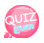# if statement

One of the conditional statements. As you program, you use it a lot. Usage is

```if(condition){
Source input to drive if conditional expression is true
}```

Let's see an example and understand.

The contents of the source are the values ​​of a if the value of variable a is 100. Outputs a string called

```<!DOCTYPE html>
<html>
<meta charset="utf-8" />
<title>JavaScript</title>
<script>
a = 100;
if(a == 100){
document.write("a has a value of 100.");
}
</script>
<body>
</body>
</html>```

Use == (= twice) when using the same condition in an if statement.
Check if the values ​​are the same and if it is true, it is executed or not.

### else if statement

The else if statement is used to assert another condition if the condition is not true in the above if statement.
So it is already paired with the if statement we wrote earlier.
In the conditional statement above, if a is 100, if a is 20, the condition is out of condition. Then if another condition a is 20, we can add more conditions.
Let's check with an example.

```<!DOCTYPE html>
<html>
<meta charset="utf-8" />
<title>JavaScript</title>
<script>
a = 20;
if(a == 100){
document.write("ifstatement works <br />");
document.write("the value of a is 100<br />");
}
else if(a == 20){
document.write("else if statement works <br />");
document.write("the value of a is 20<br />");
}
</script>
<body>
</body>
</html>```

else if문은 여러번 사용이 가능 합니다. 다른 조건이 또 필요하면 사용하면 됩니다.

### else

condition of if statement else If conditional statement of if is not, declare else and put the statement.
In other words, do this if none of the conditions are met.
Let's check with an example.

```<!DOCTYPE html>
<html>
<meta charset="utf-8" />
<title>JavaScript</title>
<script>
a = 30;
if(a == 100){
document.write("ifstatement works <br />");
document.write("the value of a is 100<br />");
}
else if(a == 20){
document.write("else if statement works <br />");
document.write("the value of a is 20<br />");
}
else{
document.write("else statement works <br />");
document.write("The value of a is neither 20 nor 100. <br />");
}
</script>